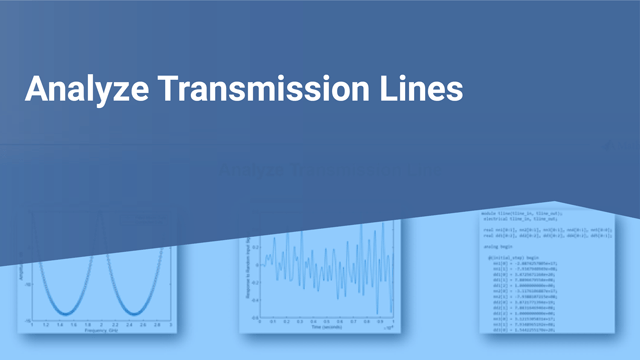# Analyze Transmission Line

In this example, you use the RF Toolbox™ command-line interface to model the time-domain response of a parallel plate transmission line. You analyze the network in the frequency domain, compute and plot the time-domain response of the network, and export a Verilog-A model of the transmission line for use in system-level simulations.

### Build and Simulate Transmission Line

Type the following command at the MATLAB® prompt to create a circuit (`rfckt`) object to represent the transmission line, which is 0.1 meters long and 0.05 meters wide:

`tline = rfckt.parallelplate('LineLength',0.1,'Width',0.05);`

Type the following set of commands at the MATLAB prompt to define the range of frequencies over which to analyze the transmission line and then run the analysis:

```f = 1.0e9:1e7:2.9e9; analyze(tline,f);```

### Compute Transmission Line Transfer Function and Time-Domain Response

This part of the example illustrates how to perform the following tasks:

• Calculate the Transfer Function

• Fit and Validate the Transfer Function Model

• Compute and Plot the Time-Domain Response

#### Calculate Transfer Function

Type the following command at the MATLAB prompt to extract the computed S-parameter values and the corresponding frequency values for the transmission line:

`[S_Params, Freq] = extract(tline,'S_Parameters');`

Type the following command at the MATLAB prompt to compute the transfer function from the frequency response data using the `s2tf` function:

`TrFunc = s2tf(S_Params);`

#### Fit and Validate Transfer Function Model

In this part of the example, you fit a rational function model to the transfer function. The toolbox stores the fitting results in an `rfmodel` object. You use the RF Toolbox `freqresp` method to validate the fit of the rational function model.

Type the following command at the MATLAB prompt to fit a rational function to the computed data and store the result in an `rfmodel` object:

`RationalFunc = rationalfit(Freq,TrFunc)`
```RationalFunc = rfmodel.rational with properties: A: [7x1 double] C: [7x1 double] D: 0 Delay: 0 Name: 'Rational Function' ```

Type the following command at the MATLAB prompt to compute the frequency response of the fitted model data:

`[fresp,freq] = freqresp(RationalFunc,Freq);`

Type the following set of commands at the MATLAB prompt to plot the amplitude of the frequency response of the fitted model data and that of the computed data:

```figure plot(freq/1e9,20*log10(abs(fresp)),freq/1e9,20*log10(abs(TrFunc))) xlabel('Frequency, GHz') ylabel('Amplitude, dB') legend('Fitted Model Data','Computed Data')```The amplitude of the model data is very close to the amplitude of the computed data. You can control the tradeoff between model accuracy and model complexity by specifying the optional tolerance argument, `tol`, to the `rationalfit` function, as described in Export Verilog-A Model.

Type the following set of commands at the MATLAB prompt to plot the phase angle of the frequency response of the fitted model data and that of the computed data:

```figure plot(freq/1e9,unwrap(angle(fresp)),... freq/1e9,unwrap(angle(TrFunc))) xlabel('Frequency, GHz') ylabel('Phase Angle, radians') legend('Fitted Data','Computed Data')```The phase angle of the model data is very close to the phase angle of the computed data.

#### Compute and Plot Time-Domain Response

In this part of the example, you compute and plot the time-domain response of the transmission line.

Type the following set of commands at the MATLAB prompt to create a random input signal and compute the time response, `tresp`, of the fitted model data to the input signal:

```SampleTime = 1e-12; NumberOfSamples = 1e4; OverSamplingFactor = 25; InputTime = double((1:NumberOfSamples)')*SampleTime; InputSignal = ... sign(randn(1, ceil(NumberOfSamples/OverSamplingFactor))); InputSignal = repmat(InputSignal, [OverSamplingFactor, 1]); InputSignal = InputSignal(:); [tresp,t] = timeresp(RationalFunc,InputSignal,SampleTime);```

Type the following set of commands at the MATLAB prompt to plot the time response of the fitted model data:

```figure plot(t,tresp) xlabel('Time (seconds)') ylabel('Response to Random Input Signal')```#### Export Verilog-A Model

In this part of the example, you export a Verilog-A model of the transmission line. You can use this model in other simulation tools for detailed time-domain analysis and system simulations.

The following code illustrates how to use the `writeva` method to write a Verilog-A module for `RationalFunc` to the file `tline.va`. The module has one input, `tline_in`, and one output, `tline_out`. The method returns a `status` of `True`, if the operation is successful, and `False` if it is unsuccessful.

`status = writeva(RationalFunc,'tline','tline_in','tline_out')`
```status = logical 1 ```

For more information on the `writeva` method and its arguments, see the `writeva` reference page.